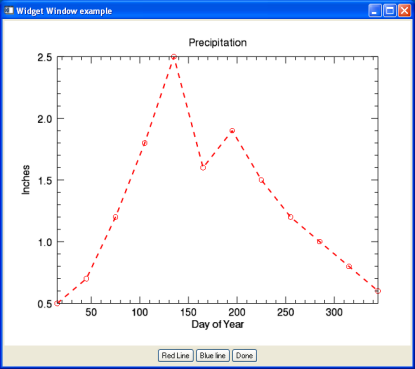You can combine graphics within IDL widget applications by using the WIDGET_WINDOW function. Adding graphics to widget applications gives you the functionality of interactive graphic window with the ability to add your own GUI elements.The code shown below creates the GUI (widget) application above. You can copy the entire block and paste it into the IDL editor, or enter .edit plot_widget_doc at the IDL command line to open the program.

`PRO PLOT_WIDGET_DOC_EVENT, event`
` `
`  CASE TAG_NAMES(event, /STRUCTURE_NAME) OF`
`    'WIDGET_BUTTON': BEGIN`
`    WIDGET_CONTROL, event.id, GET_UVALUE = event_UV`
` `
`    ; Retrieve the Widget Window`
`    wDraw = WIDGET_INFO(event.top, FIND_BY_UNAME = 'DRAW')`
`    WIDGET_CONTROL, wDraw, GET_VALUE = graphicWin`
` `
`    ; Retrieve the plot with the NAME`
`    ; provided on plot creation`
`    p = graphicWin['PLOT_WIDGET_DOC']`
`    CASE event_UV OF`
`      'DONE': WIDGET_CONTROL, event.top, /DESTROY`
`      'RED': p.color='red'`
`      'BLUE': p.color='blue'`
`      ELSE: ; do nothing`
`    ENDCASE`
`    END`
` `
`    'WIDGET_BASE': begin`
`    ; Handle base resize events. Retrieve our cached padding,`
`    ; and our new size.`
`    WIDGET_CONTROL, event.id, GET_UVALUE=pad, TLB_GET_SIZE=newSize`
`    wDraw = WIDGET_INFO(event.top, FIND_BY_UNAME='DRAW')`
`    ; Change the draw widget to match the new size, minus padding.`
`    xy = newSize - pad`
`    WIDGET_CONTROL, wDraw, \$`
`    DRAW_XSIZE=xy, DRAW_YSIZE=xy, \$`
`    SCR_XSIZE=xy, SCR_YSIZE=xy`
`    end`
`  `
`    ELSE: ; do nothing`
`  ENDCASE`
`END`
` `
`PRO PLOT_WIDGET_DOC`
`  base1 = WIDGET_BASE(/COLUMN, TITLE='Widget Window example', \$`
`    /TLB_SIZE_EVENTS)`
` `
`  wDraw = WIDGET_WINDOW(base1, UVALUE='draw', UNAME='DRAW')`
` `
`  ; Create the base for the button:`
`  base2 = WIDGET_BASE(base1, /ROW, /ALIGN_CENTER)`
` `
`  ; Create the action buttons.`
`  redline = WIDGET_BUTTON(base2, VALUE='Red Line', UVALUE = 'RED')`
`  blueline = WIDGET_BUTTON(base2, VALUE='Blue line', UVALUE='BLUE')`
`  done = WIDGET_BUTTON(base2, VALUE = 'Done', UVALUE = 'DONE')`
` `
`  ; Realize the widget (i.e., display it on screen).`
`  WIDGET_CONTROL, base1, /REALIZE`
` `
`  ; Register the widget with the XMANAGER, leaving the IDL command`
`  ; line active.`
`  XMANAGER, 'PLOT_WIDGET_DOC', base1, /NO_BLOCK`
` `
`  ; Cache the padding between the base and the draw`
`  WIDGET_CONTROL, base1, TLB_GET_SIZE=basesize`
`  xpad = basesize - 640`
`  ypad = basesize - 512`
`  WIDGET_CONTROL, base1, SET_UVALUE=[xpad,ypad]`
` `
`  ; Retrieve the newly-created Window object.`
`  WIDGET_CONTROL, wDraw, GET_VALUE = graphicWin`
` `
`  graphicWin.SELECT`
` `
`  PRECIP=[0.5,0.7,1.2,1.8,2.5,1.6,1.9,1.5,1.2,1.0,0.8,0.6]`
`  TEMP=[30, 34, 38, 47, 57, 67, 73, 71, 63, 52, 39, 33]`
`  DAY=FINDGEN(12) * 30 + 15`
` `
`  ; Plot #1: In position #1 on the grid defined by LAYOUT`
`  p=PLOT(DAY, PRECIP, NAME = 'PLOT_WIDGET_DOC', SYMBOL='o', \$`
`    LINESTYLE='--', TITLE = 'Precipitation', \$`
`    YTITLE = 'Inches', XTITLE= 'Day of Year', \$`
`    THICK=2, /CURRENT)`
`END`